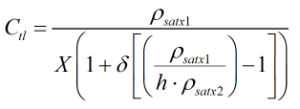# C254 – API MPMS/GPA TP-27 Ctl Calculation

## Description

This calculates the temperature correction factor for the volume of natural gas liquids and liquefied petroleum gases according to the TP-27 specification.

GPA Technical Publication TP-27, ASTM MPMS 11.2.4 – Temperature Correction for the Volume of NGL and LPG Tables 23E, 24E, 53E, 54E 59E and 60E

Kelton calculation reference C254

This calculation is used by:

F064/K213 – API MPMS/GPA TP-27:2007 Density Referral

## Options

### Standard Temperature

• 15°C
• 20°C
• 60°F

This option allows the user to select which standard conditions they are using as a reference.

### Solve For

• Density
• Standard density

This option is used to select the desires output of the calculation.

### Precision

• Full precision
• Rounding

### Density Input

• Enter Density as Relative Density

## Constants

Density of water at 60°F ρw60 = 999.016 kgm-3

Table 1: Reference Fluid Parameters

Fluid Name γ60 Zc Tc ρc k1 k2 k3 k4
EE(68/32) 0.325022 0.279980 298.11 6.250 2.54616855327 -0.058244177754 0.803398090807 -0.745720314137
Ethane 0.355994 0.282200 305.33 6.870 1.89113042610 -0.370305782347 -0.544867288720 0.337876634952
EP(65/35) 0.429277 0.280600 333.67 5.615 2.20970078464 -0.294253708172 -0.405754420098 0.319443433421
EP(35/65) 0.470381 0.279300 352.46 5.110 2.25341981320 -0.266542138024 -0.372756711655 0.384734185665
Propane 0.507025 0.276260 369.78 5.000 1.96568366933 -0.327662435541 -0.417979702538 0.303271602831
i-Butane 0.562827 0.283260 407.85 3.860 2.04748034410 -0.289734363425 -0.330345036434 0.291757103132
n-Butane 0.584127 0.275360 425.16 3.920 2.03734743118 -0.299059145695 -0.418883095671 0.380367738748
i-Pentane 0.624285 0.270260 460.44 3.247 2.06541640707 -0.238366208840 -0.161440492247 0.258681568613
n-Pentane 0.631054 0.272350 469.65 3.200 2.11263474494 -0.261269413560 -0.291923445075 0.308344290017
i-Hexane 0.657167 0.267060 498.05 2.727 2.02382197871 -0.423550090067 -1.152810982570 0.950139001678
n-Hexane 0.664064 0.267620 507.35 2.704 2.17134547773 -0.232997313405 -0.267019794036 0.378629524102
n-Heptane 0.688039 0.263120 540.15 2.315 2.19773533433 -0.275056764147 -0.447144095029 0.493770995799

## Calculation

The Ctl is calculated by using an iterative process unless the standard temperature is 60°F. The iterative regime converts the standard density to the relative density at 60°F. The calculation works by referencing this relative density value against the properties of 2 reference fluids at a standard temperature of 60°F. An algorithm determines which reference fluids should be used according to the relative density of the input fluid. A further algorithm then calculates the Ctl from a series of relationships stemming from the reference fluid parameters.Where h = Scaling factor δ = Interpolation variable X = Interpolating factor ρsat601 = Saturation density of reference fluid 1 at 60°F ρsat602 = Saturation density of reference fluid 2 at 60°F ρsatx1 = Saturation density of reference fluid 1 at reduced observed temperature ρsatx2 = Saturation density of reference fluid 2 at reduced observed temperature

Back